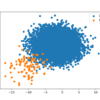# Archive | Imbalanced Classification## How to Fix k-Fold Cross-Validation for Imbalanced Classification

Model evaluation involves using the available dataset to fit a model and estimate its performance when making predictions on unseen examples. It is a challenging problem as both the training dataset used to fit the model and the test set used to evaluate it must be sufficiently large and representative of the underlying problem so […]## A Gentle Introduction to Probability Metrics for Imbalanced Classification

Classification predictive modeling involves predicting a class label for examples, although some problems require the prediction of a probability of class membership. For these problems, the crisp class labels are not required, and instead, the likelihood that each example belonging to each class is required and later interpreted. As such, small relative probabilities can carry […]## Tour of Evaluation Metrics for Imbalanced Classification

A classifier is only as good as the metric used to evaluate it. If you choose the wrong metric to evaluate your models, you are likely to choose a poor model, or in the worst case, be misled about the expected performance of your model. Choosing an appropriate metric is challenging generally in applied machine […]## ROC Curves and Precision-Recall Curves for Imbalanced Classification

Most imbalanced classification problems involve two classes: a negative case with the majority of examples and a positive case with a minority of examples. Two diagnostic tools that help in the interpretation of binary (two-class) classification predictive models are ROC Curves and Precision-Recall curves. Plots from the curves can be created and used to understand […]## How to Calculate Precision, Recall, and F-Measure for Imbalanced Classification

Classification accuracy is the total number of correct predictions divided by the total number of predictions made for a dataset. As a performance measure, accuracy is inappropriate for imbalanced classification problems. The main reason is that the overwhelming number of examples from the majority class (or classes) will overwhelm the number of examples in the […]## Failure of Classification Accuracy for Imbalanced Class Distributions

Classification accuracy is a metric that summarizes the performance of a classification model as the number of correct predictions divided by the total number of predictions. It is easy to calculate and intuitive to understand, making it the most common metric used for evaluating classifier models. This intuition breaks down when the distribution of examples […]## Standard Machine Learning Datasets for Imbalanced Classification

An imbalanced classification problem is a problem that involves predicting a class label where the distribution of class labels in the training dataset is skewed. Many real-world classification problems have an imbalanced class distribution, therefore it is important for machine learning practitioners to get familiar with working with these types of problems. In this tutorial, […]## Develop an Intuition for Severely Skewed Class Distributions

An imbalanced classification problem is a problem that involves predicting a class label where the distribution of class labels in the training dataset is not equal. A challenge for beginners working with imbalanced classification problems is what a specific skewed class distribution means. For example, what is the difference and implication for a 1:10 vs. […]## Best Resources for Imbalanced Classification

Classification is a predictive modeling problem that involves predicting a class label for a given example. It is generally assumed that the distribution of examples in the training dataset is even across all of the classes. In practice, this is rarely the case. Those classification predictive models where the distribution of examples across class labels […]## A Gentle Introduction to Imbalanced Classification

Classification predictive modeling involves predicting a class label for a given observation. An imbalanced classification problem is an example of a classification problem where the distribution of examples across the known classes is biased or skewed. The distribution can vary from a slight bias to a severe imbalance where there is one example in the […]